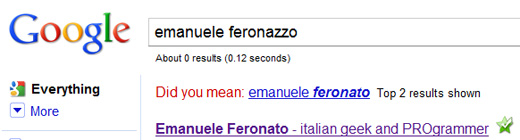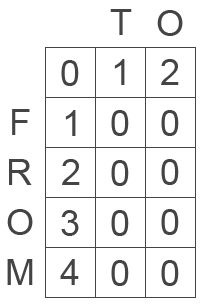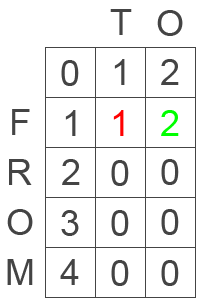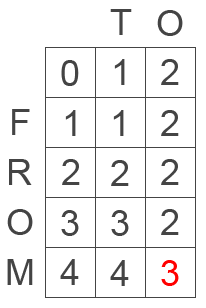# Find Levenshtein distance with AS3

From Wikipedia: The Levenshtein distance between two strings is defined as the minimum number of edits needed to transform one string into the other, with the allowable edit operations being insertion, deletion, or substitution of a single character.

In real world, one of the most common uses of this distance is the suggestion Google throws when it thinks you mispelled your search.When you search for an uncommon word that is similar to a popular search, Google suggests the word it supposes you were looking for.

This is probably made with Levenshtein distance. Some languages such as PHP have their native function to determine the distance between two string, but Actionscript 3 does not have any function to accomplish this task.

That’s why I am showing you how to do it

The Levenshtein algorithm

Giving two string made by respectively `n` and `m` characters, we create a `m`*`n` matrix this way:This is the starting matrix, with all cells close to the strings set at their `n`-th character and the remaining ones at zero.

Then, we must fill the matrix from the upper left to the lower right corner starting from cell `1`,`1` (second row, second column) in this way:

I fill the `x`,`y` cell, with the minimum value among

* `x-1`,`y` cell value + `1`
* `x`,`y-1` cell value + `1`
* `x-1`,`y-1` cell value + `d`

where `d` = `0` if the `x`th character of the second string is the same as the `y`th character of the first string, and `1` if such characters are different.

So in our case the first line of the matrix will be filled this way:The red value is determined by the minimum value among `2` (leftmost cell value plus one), `2` (upper cell value plus one) and `1` (upper left diagonal cell value plus one because `T` and `F` aren’t the same character)

The green value is determined by the minimum value among `2` (leftmost cell value plus one), `2` (upper cell value plus one) and `2` (upper left diagonal cell value plus one because `O` and `F` aren’t the same character)

Apply this principle to the entire matrix and you will getThe red number at the lower right of the matrix is the distance.

Here it is translated into AS3:

```package {
import flash.display.Sprite;
import flash.text.TextField;
import flash.text.TextFieldType;
import flash.text.TextFormat;
import flash.events.Event;
public class levenshtein extends Sprite {
var from:TextField=new TextField();
var to:TextField=new TextField();
var lev:TextField=new TextField();
var text_format:TextFormat = new TextFormat();
public function levenshtein():void {
text_format.color=0x000000;
text_format.size=24;
with (from) {
type=TextFieldType.INPUT;
x=20;
y=20;
width=460;
height=30;
text="from";
border=true;
setTextFormat(text_format);
}
with (to) {
type=TextFieldType.INPUT;
x=20;
y=70;
width=460;
height=30;
text="to";
border=true;
setTextFormat(text_format);
}
with (lev) {
x=20;
y=120;
width=460;
height=30;
text="distance = "+distance(from.text,to.text);
setTextFormat(text_format);
}
}
public function on_change(e:Event):void {
lev.text="distance = "+distance(from.text,to.text);
lev.setTextFormat(text_format);
}
public function distance(string_1:String, string_2:String):int {
var matrix:Array=new Array();
var dist:int;
for (var i:int=0; i<=string_1.length; i++) {
matrix[i]=new Array();
for (var j:int=0; j<=string_2.length; j++) {
if (i!=0) {
matrix[i].push(0);
} else {
matrix[i].push(j);
}
}
matrix[i]=i;
}
for (i=1; i<=string_1.length; i++) {
for (j=1; j<=string_2.length; j++) {
if (string_1.charAt(i-1)==string_2.charAt(j-1)) {
dist=0;
} else {
dist=1;
}
matrix[i][j]=Math.min(matrix[i-1][j]+1,matrix[i][j-1]+1,matrix[i-1][j-1]+dist);
}
}
return matrix[string_1.length][string_2.length];
}
}
}```

And this is the result:

Type anything you want in both text areas and see the Levenshtein distance in real time

214 GAME PROTOTYPES EXPLAINED WITH SOURCE CODE
// 1+2=3
// 10000000
// 2 Cars
// 2048
// Avoider
// Ballz
// Block it
// Blockage
// Bloons
// Boids
// Bombuzal
// Breakout
// Bricks
// Columns
// CubesOut
// Dots
// DROP'd
// Dudeski
// Eskiv
// Filler
// Fling
// Globe
// HookPod
// Hundreds
// InkTd
// Iromeku
// Lumines
// Magick
// MagOrMin
// Maze
// Memdot
// Nano War
// Nodes
// o:anquan
// Ononmin
// Pacco
// Phyballs
// Platform
// Poker
// Pool
// Poux
// Pudi
// qomp
// Racing
// Renju
// SameGame
// Security
// Sling
// Slingy
// Sokoban
// Splitter
// Sproing
// Stack
// Stringy
// Sudoku
// Tetris
// Threes
// Toony
// Turn
// TwinSpin
// vvvvvv
// Wordle
// Worms
// Yanga
// Zhed
// zNumbers